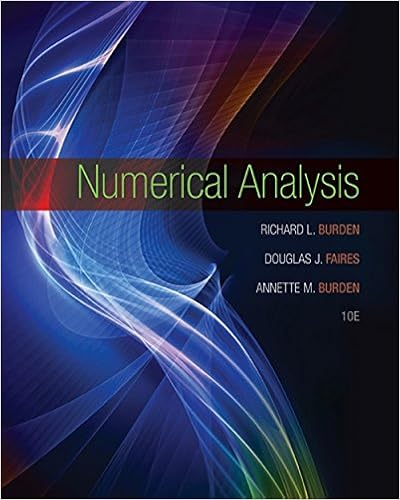# 62 rounding and machine precision to represent a real

• 213
• 100% (3) 3 out of 3 people found this document helpful

This preview shows page 109 - 112 out of 213 pages.

##### We have textbook solutions for you!
The document you are viewing contains questions related to this textbook.The document you are viewing contains questions related to this textbook.
Chapter 4 / Exercise 7
Numerical Analysis
Burden/FairesExpert Verified
6.2 Rounding and Machine Precision To represent a real number x as a floating point number, rounding has to be performed to retain only the numbers of binary bits allowed in the significant. Let x R and its binary expansion be x = ± (1 .b 1 b 2 · · · ) 2 × 2 E . One way to approximate x to a floating number with d bits in the signif- icant is to truncate or chop discarding all the bits after b d , i.e. x * = chop( x ) = ± (1 .b 1 b 2 · · · b d ) 2 × 2 E . (6.5) In double precision d = 52. A better way to approximate to a floating point number is to do rounding up or down (to the nearest floating point number), just as we do when we round in base 10. In binary, rounding is simpler because b d +1 can only be 0 (we round down) or 1 (we round up). We can write this type of rounding in terms of the chopping described above as x * = round( x ) = chop( x + 2 - ( d +1) × 2 E ) . (6.6) Definition 9. Given an approximation x * to x the absolute error is defined by | x - x * | and the relative error by | x - x * x | , x 6 = 0 . The relative error is generally more meaningful than the absolute error to measure a given approximation.
##### We have textbook solutions for you!
The document you are viewing contains questions related to this textbook.The document you are viewing contains questions related to this textbook.
Chapter 4 / Exercise 7
Numerical Analysis
Burden/FairesExpert Verified
6.3. CORRECTLY ROUNDED ARITHMETIC 101 The relative error in chopping and in rounding (called a round-off error ) is x - chop( x ) x 2 - d 2 E (1 .b 1 b 2 · · · )2 E 2 - d , (6.7) x - round( x ) x 1 2 2 - d . (6.8) The number 2 - d is called machine precision or epsilon (eps). In double precision eps=2 - 52 2 . 22 × 10 - 16 . The smallest double precision number greater than 1 is 1+eps. As we will see below, it is more convenient to write (6.8) as round( x ) = x (1 + δ ) , | δ | ≤ eps . (6.9) 6.3 Correctly Rounded Arithmetic Computers today follow the IEEE standard for floating point representation and arithmetic. This standard requires a consistent floating point represen- tation of numbers across computers and correctly rounded arithmetic . In correctly rounded arithmetic, the computer operations of addition, sub- traction, multiplication, and division are the correctly rounded value of the exact result . If x and y are floating point numbers and is the machine addition, then x y = round( x + y ) = ( x + y )(1 + δ + ) , | δ + | ≤ eps , (6.10) and similarly for , , . One important interpretation of (6.10) is the the following. Assuming x + y 6 = 0, write δ + = 1 x + y [ δ x + δ y ] . Then x y = ( x + y ) 1 + 1 x + y ( δ x + δ y ) = ( x + δ x ) + ( y + δ y ) . (6.11) The computer is giving the exact result but for a sightly perturbed data . This interpretation is the basis for Backward Error Analysis , which is used to study how round-off errors propagate in a numerical algorithm.
102 CHAPTER 6. COMPUTER ARITHMETIC 6.4 Propagation of Errors and Cancellation of Digits Let fl ( x ) and fl ( y ) denote the floating point approximation of x and y , respectively, and assume that their product is computed exactly, i.e fl ( x ) · fl ( y ) = x (1+ δ x ) · y (1+ δ y ) = x · y (1+ δ x + δ y + δ x δ y ) x · y (1+ δ x + δ y ) , where | δ x | , | δ y | ≤ eps. Therefore, for the relative error we get x · y - fl ( x ) · fl ( y ) x · y ≈ | δ x + δ y | , (6.12) which is acceptable.
•••